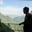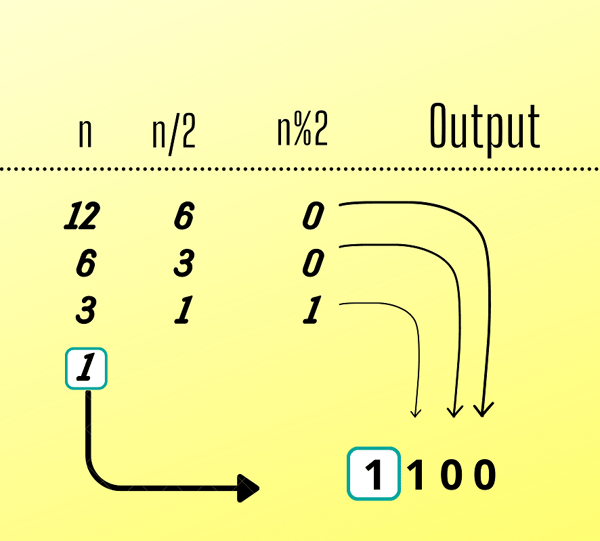Related Tags

recursion
communitycreator

# How to convert a decimal to binary through recursionAKASH BAJWA

Recursion is when a function indirectly or directly calls itself. Humans count in base 10; in contrast, computers count in base 2 because of the binary nature of electronics. Now, we are going to use the recursion method for this problem.Function Call (Unstacking Calls)

### Stopping conditions

Let’s consider an example where a programmer wants to compute the sum of the first $N$ numbers. There are multiple ways to do this, but we will follow a recursive method, so the function will be as follows:

//Function Call:   //Base Case:
f(n) = 1             n=1
f(n) = n + f(n-1)    n>1


### Demo code

There are only two cases to discuss in line 11 in the Java program.

• One is a base case: if (n == 0)
• The other is a general case: return (n % 2 + 10 * getBinary(n / 2))

Recursion is always terminated by the base case.

// Java program to demonstrate the working of
// recursion

class Edpresso {
public static int getBinary(int n) // generate Binary
{

if (n == 0)
return 0;

return (n % 2 + 10 * getBinary(n / 2));
}

// Driver Code
public static void main(String[] args)
{
int N = 6; // Number to find
System.out.print(N + " in Binary: " + getBinary(N));
}
}


RELATED TAGS

recursion
communitycreator

CONTRIBUTORAKASH BAJWA
RELATED COURSES

View all Courses

Keep Exploring

Learn in-demand tech skills in half the time